# Cuboid Problems

A cuboid is a three-dimensional shape with a length, width, and height. A cuboid is a Rectangular Prism. The cuboid body has six sides called faces. Each face of a cuboid is a rectangle, and all of a cuboid's corners (called vertices) are 90-degree angles. Opposite faces are parallel. A cuboid has the shape of a rectangular box.

#### Number of problems found: 326

• ThereThere is water in the block-shaped tank with dimensions of 3 m 1.5 m 5 m, it occupies 70% of the tank volume. Find the volume of water in the tank (in hl) .
• Swimming pool 5A rectangular inflatable swimming pool is 3 yards long, 2 5/8 yards wide, and  1 3/5 yard tall. What is the volume of the pool? Round to the nearest tenth.
• An aquariumAn aquarium tank that measures 2.4 m high, 6 m long, and 1.5 m wide and is completely filled with water. The density of water is 1,000 kg/m3. What is the mass of the water in the tank?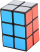A gift box has dimensions of: 8 1/2 inches, 5 1/2 inches, and 2 1/2 inches, respectively. How many cubes with side lengths of 1/2 inches would be needed to fill the gift box?
• An architectAn architect is designing a house. He wants the bedroom to have the dimensions of 8 ft by 4 ft by 7 ft. The architect doubles all three dimensions to create the den. Does that mean the den will have double the volume of the bedroom? First, find the volume
• Bathtub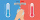Kevin has a bathtub shaped like a block. The bathtub measures 70 cm long, 45 cm wide and 50 cm high. Then the bathtub will be filled with clean water 3/5 of its height. The clean water needed is….
• A dumpsterA dumpster is shaped like a rectangular prism. It has a width of 8 feet, a height of 5 feet, and a volume of 880 cubic feet. What is the length of the dumpster?
• Water overflow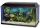A rectangular container that has a length of 30 cm, a width of 20 cm, and a height of 24 cm is filled with water to a depth of 15 cm. When an additional 6.5 liters of water are poured into the container, some water overflows. How many liters of water over
• Mr. Franklin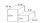Mr. Franklin built a new set of stairs to his side door as shown. What is the total volume of Mr. Franklin’s new set of stairs?
• Edges of the cuboid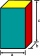Find the length of the edges of the cuboid, which has the following dimensions: width is 0.4 m; the height is 5.8 dm and the block can hold 81.2 liters of fluid.
• What power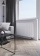What power should the radiator have in a room 5x4 meters with a height of 3 meters? Hint: Calculate 35 watts per meter ^ 3 in case you need 22 degrees in the room but you need about 24 degrees in the bathroom. It's an old heating cheat, but it will defini
• A butterA butter cube with an edge 6.5 cm long is packed in a package with dimensions a = 28 cm, b = 15 cm. Calculate how many cm2 the package is larger than the surface of the cube.
• Dana helped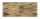Dana helped her dad build a sandbox for her younger sister. The sandbox is shaped like a rectangular prism that is 4 1/2 feet long and 4 feet wide. Dana used bags of sand to fill the sandbox 1/2 of a foot deep. Each bag contained 1/2 of a cubic foot of sa
• Waste container eco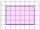The waste box has the shape of a block with dimensions of 1.8 m, 1.5 m, 1.2 m. 0.25 m3 of waste is added to it every day. How many days will it be filled?
• Empty aquariumHow much does an empty aquarium weigh with dimensions: length = 40 cm, width = 30 cm, height = 20 cm, if 1 dm2 of glass weighs 300 g? Calculate its weight in kilograms.
• Regular square prismThe volume of a regular square prism is 192 cm3. The size of its base edge and the body height is 1: 3. Calculate the surface of the prism.
• An architect 2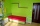An architect is designing a house. He wants the bedroom to have the dimensions of 8 ft by 4 ft by 7 ft. The architect doubles all three dimensions to create the den. Does that mean the den will have double the volume of the bedroom? First, find the volume
• A box 2A box has a length of 4 1/2 inches, a width of 3 2/3 inches and a height of 8 1/4 inches. What is the volume of the box?
• Consider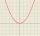Consider all square prisms with a height of 10 cm. If x is the measurement of the base edge, in cm, and y is the volume of the prism, in cm3. Graph the function
• The squareThe square oak board (with density ρ = 700 kg/m3) has a side length of 50 cm and a thickness of 30 mm. 4 holes with a diameter of 40 mm are drilled into the board. What is the weight of the board?

Do you have an exciting math question or word problem that you can't solve? Ask a question or post a math problem, and we can try to solve it.

We will send a solution to your e-mail address. Solved examples are also published here. Please enter the e-mail correctly and check whether you don't have a full mailbox.

Please do not submit problems from current active competitions such as Mathematical Olympiad, correspondence seminars etc...